## Mathematical Notation

For various reasons, it has been necessary to include some less complicated mathematical equations (such as x^2+y^2=r_0) directly in the text. Due to the limitations of some terminals and the HTML language, some notation has been used in these equations that may not be familiar to you. This page will attempt to explain all such notation.

Relational symbols likemay be represented in text equations as <= and >=, respectively. Any greek letters such as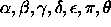will be spelled out in a text equation (i.e. "the circumference of a circle is C=2(pi)r"). In a line of text exponentiation will be indicated by using the caret symbol "^". For instance, the expression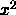for the quantity "x squared" will be written as x^2 in text. In a somewhat similar vein, the exponential functionmay be written as e^x or exp(x).

These pages assume you are familiar with the standard transcendental functions, including the exponential function ("exp"), the natural logarithm ("ln" or "log") and the six trigonometric functions ("sin", "cos", "tan", "cot", "sec", and "csc").

Subscripts have two different notations in these pages. In the material on sequences and series, the notation a[n] represents the nth term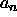in the sequence or series. In many other pages, subscripts will be denoted with an underscore. For instance, a point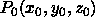will often be written as P_0(x_0,y_0,z_0).

These pages use the symbol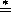to indicate where l'Hôpital's Rule is being used, as opposed to algebraic manipulation or basic limit facts.

Derivatives are denoted by the usual "prime" notation, until you get past the third derivative, when the parenthetical notation is used. For instance, the first derivative of f(x) with respect to x has the consistent notation f '(x) whereas the fourth derivative of f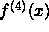is denoted f^(4)(x).